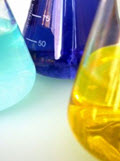# Mathematical Models 2

How to translate from English to Mathematics

## The Real World

Most mathematics questions a student gets are neat and pure and simple.

But the real world is often "messy": the numbers don't always work out nicely, we have to convert to different units, realities get in the way, and so on.

On Mathematical Models we showed how to make and use some simple models.

Here are some more.

## Example: River Cruise

### Example: a 3 hour river cruise goes 15 km upstream and then back again. The river has a current of 2 km an hour. What is the boat's speed and how long was the upstream journey?There are two speeds to think about: the speed the boat makes in the water, and the speed relative to the land:

• Let b = the boat's speed in the water (km/h)
• Let v = the speed relative to the land (km/h)

Because the river flows downstream at 2 km/h:

• when going upstream, v = b-2 (its speed is reduced by 2 km/h)
• when going downstream, v = b+2 (its speed is increased by 2 km/h)

We can turn those speeds into times using:

time = distance / speed

(moving 8 km at 4 km/h takes 8/4 = 2 hours, right?)

And we know the total time is 3 hours:

total time = 3 hours = time upstream + time downstream

Put all that together:

total time = 3 hours = 15/(b−2) + 15/(b+2)

Now we use our algebra skills to solve for "b". Start with:

3 = 15/(b−2) + 15/(b+2)

Get rid of the fractions by multiply through by (b-2)(b+2):

3(b2)(b+2) = 15(b+2) + 15(b2)

Expand everything:

3(b2−4)= 15b+30 + 15b−30

Bring everything to the left and simplify:

3b2 − 30b − 12 = 0

It is a Quadratic Equation! And solving it gives the solutions:

b = −0.39 or 10.39 (to 2 decimal places)

• b = −0.39 makes no sense for this real world question,
• but b = 10.39 is just perfect!

Answer: Boat's Speed = 10.39 km/h (to 2 decimal places)

And so the upstream journey = 15 / (10.39−2) = 1.79 hours = 1 hour 47 min

And the downstream journey = 15 / (10.39+2) = 1.21 hours = 1 hour 13 min

## Example: Mixing Solutions

### You have been asked to provide 150 mL of hydrochloric acid at a 12% concentrationIn the cabinet you find:

• one bottle marked 10% HCl (10% hydrochloric acid)
• and another bottle marked 25% HCl (25% hydrochloric acid)

How many mL of each should you use?

• a = quantity of 10% solution
• 150 − a = quantity of 25% solution

Total HCl = HCl in 10% solution + HCl in 25% solution

(0.12)(150) = (0.10)(a) + (0.25)(150−a)

Solve: 18 = 0.1a + 37.5 − 0.25a

Put "a"s on the left, the rest to right: 0.25a − 0.1a = 37.5−18

Simplify: 0.15a = 19.5

a = 19.5/0.15 = 130

Answer: 130 ml of 10% solution and 20 ml of 25% solution

Note: We have a Mix Slider that can also be used to solve this